Our prices are denominated in USD.

We ship internationally!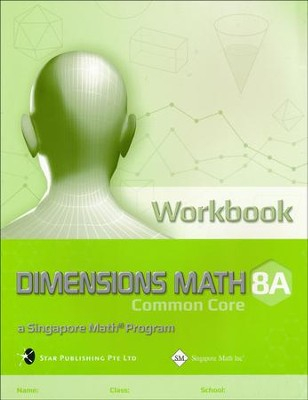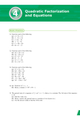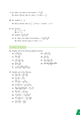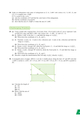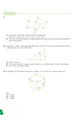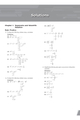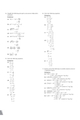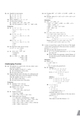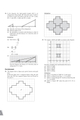# Dimensions Mathematics Common Core Workbook 8A

\$10.70

Each workbook is written as a supplement to the textbook, Dimensions Math®, to give students more practice in applying the concepts learned. Students may refer to the summary of the important concepts in each chapter of the textbook for a quick review before attempting the questions in the workbook. After completing the exercises, students will not only polish their own analytical skills, but also develop a stronger foundation in mathematics.

The questions in each workbook chapter are categorized into 4 parts according to the level of difficulty and the thinking skills involved:

• Basic Practice: simple questions that drill comprehension of concepts
• Further Practice: harder questions that involve direct applications
• Challenging Practice: questions that require synthesis ability
• Enrichment: questions that demand higher order thinking

These questions encourage students to think analytically, reason logically, use appropriate connections between mathematical ideas, and apply problem-solving skills in daily life situations.

Workbook Solutions provide all detailed worked solutions to questions in workbook.

Chapter 1: Exponents and Scientific Notation

Chapter 2: Linear Equations in Two Variables

Chapter 3: Expansion and Factorization of Algebraic Expressions

Chapter 4: Quadratic Factorization and Equations

Chapter 5: Simple Algebraic Fractions

Chapter 6: Congruence and Reflections

Chapter 7: Parallel Lines and Angles in Triangles and Polygons

Complete series contain:

1. Textbooks (A & B) - Soft cover
2. Workbooks (A & B)
3. Workbook Solutions (A & B)
4. Teacher's Notes & Solutions ( A & B)
5. Levels 6 - 8